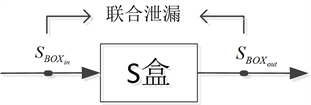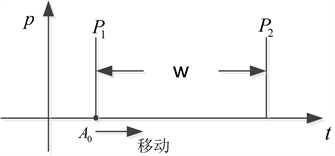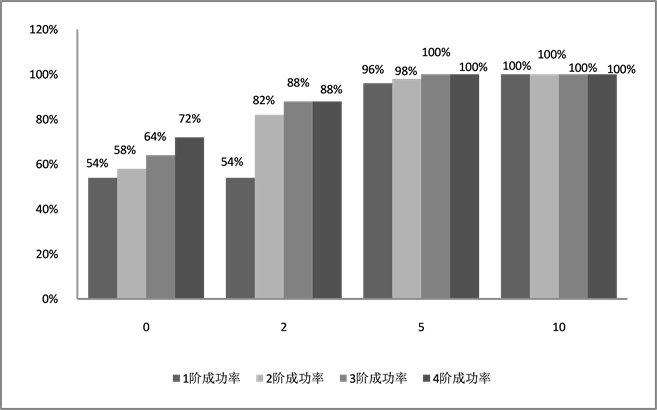# 侧信道攻击中的高阶模板攻击研究Research on High Order Template Attack in Side Channel Attack

• 全文下载: PDF(388KB)    PP.13-18   DOI: 10.12677/JSST.2018.61003
• 下载量: 789  浏览量: 2,520

In the side channel attack, the mask implementation which is used to resist the first-order DPA attack is widely used at present. There are great defects in the high-order DPA with this implementation both in the cost and rate. In order to improve the deficiencies of existing domestic and foreign attack technology, we will introduce the high-order template attacks for masked implementation and its mathematical models and algorithms. The data processing and experiment are based on Matlab platform, and the results will prove the feasibility of the method.

1. 引言

2. 模板攻击

3. 掩码对策

4. 高阶攻击

4.1. 二阶DPA攻击Figure 1. Joint leakage in energy trace diagram

${\rho }_{XY}=\frac{E\left\{\left[X-E\left(X\right)\right]\cdot \left[Y-E\left(Y\right)\right]\right\}}{\sqrt{D\left(X\right)}\cdot \sqrt{D\left(Y\right)}}$ ,

1) 假设一个P1与P2之间的间距，记为w，此时选择一个P1的起始位置A0，即可计算出相关系数P。

2) 不断向右移动P1的位置A，并将相关系数P记录下来。如图2所示。

3) 找到P的最大值，并将w减小，返回步骤1重复执行上述步骤。

4) 所有遍历结束后，挑选其中最大的相关系数P作为最终结果。

4.2. 二阶模板攻击Figure 2. 2-order DPA attack algorithm diagram

5. 实验过程及结果分析

$p\left(t;\left(m,C\right)\right)=\frac{\mathrm{exp}\left(-\frac{1}{2}\cdot \left(t-m\right)\right)\cdot {C}^{-1}\cdot \left(t-m\right)\right)}{\sqrt{{\left(2\cdot \pi \right)}^{Τ}\cdot \mathrm{det}\left(C\right)}}$ .Figure 3. 2-order DPA and 2-order template attack success rate comparison schematic diagram

$N$ ，n阶攻击成功次数记为 ${N}_{0}$ ，则n阶攻击成功率 $\eta$ 为： $\eta =\frac{{N}_{0}}{N}×100%$

6. 结论

NOTES

*通讯作者。

  Kocher, P., Jaffe, J. and Jun, B. (1999) Differential Power Analysis. Annual International Cryptology Conference, Springer, Berlin, Heidelberg, 388-397. https://doi.org/10.1007/3-540-48405-1_25  Messerges, T.S. (2000) Using Second-Order Power Analysis to Attack DPA Resistant Software. International Workshop on Cryptographic Hardware and Embedded Systems, Springer, Berlin, Heidelberg, 238-251. https://doi.org/10.1007/3-540-44499-8_19  Oswald, E., Mangard, S., Herbst, C., et al. (2006) Practical Second-Order DPA Attacks for Masked Smart Card Implementations of Block Ciphers. Cryptographers’ Track at the RSA Conference, Springer, Berlin, Heidelberg, 192-207.  Oswald, E. and Mangard, S. (2007) Template Attacks on Masking—Resistance Is Futile. Cryptographers’ Track at the RSA Conference, Springer, Berlin, Heidelberg, 243-256.  Popp, T., Mangard, S. and Oswald, E. (2007) Power Analysis Attacks and Countermeasures. IEEE Design & Test of Computers, 24, 535-543. https://doi.org/10.1109/MDT.2007.200